Next: Number Representation Up: Number Systems Previous: Number Systems

## Binary, Octal and Hexadecimal Numbers

Consider a decimal number with digits a b c. We can write abc as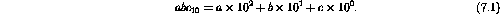Similarly, in the binary system a number with digits a b c can be written as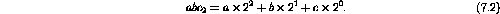Each digit is known as a bit and can take on only two values: 0 or 1. The left most bit is the highest-order bit and represents the most significant bit (MSB), while the lowest-order bit is the least significant bit (LSB).

Conversion from binary to decimal can be done using a set of rules, but it is much easier to use a calculator or tables (table 7.1).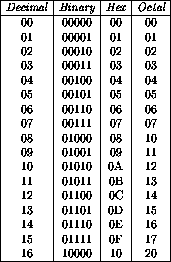Table 7.1:  Decimal, binary, hexadecimal and octal equivalents.

The eight octal numbers are represented with the symbols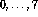, while the 16 hexadecimal numbers use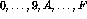.

In the octal system a number with digits a b c can be written as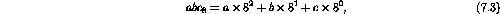while one in the hexadecimal system is written as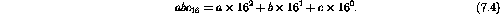A binary number is converted to octal by grouping the bits in groups of three, and converted to hexadecimal by grouping the bits in groups of four. Octal to hexadecimal conversion, or visa versa, is most easily performed by first converting to binary.

Example: Convert the binary number 1001 1110 to hexadecimal and to decimal.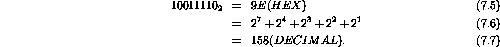Example: Convert the octal number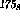to hexadecimal.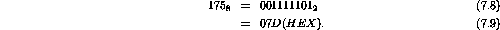Example: Convert the number 146 to binary by repeated subtraction of the largest power of 2 contained in the remaining number.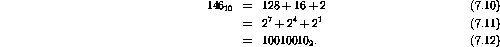Example: Devise a method similar to that used in the previous problem and convert 785 to hexadecimal by subtracting powers of 16.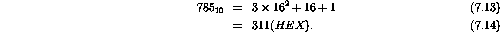Doug Gingrich
Tue Jul 13 16:55:15 EDT 1999